# Operations with Matrices [Mathematics]

Note: This tutorial mainly targets A/L students.

In this tutorial I’ll be teaching you the most common operations used with matrices and how-to remember them without much difficulty (matrices can be very complicated, you know!).

6 commonly used matrix operations

• The Inverse of a Matrix,
• The Square of a Matrix,
• Multiplying different Matrices by one another,
• Multiplying a Matrix by a number (x),
• Subtracting Matrices.
•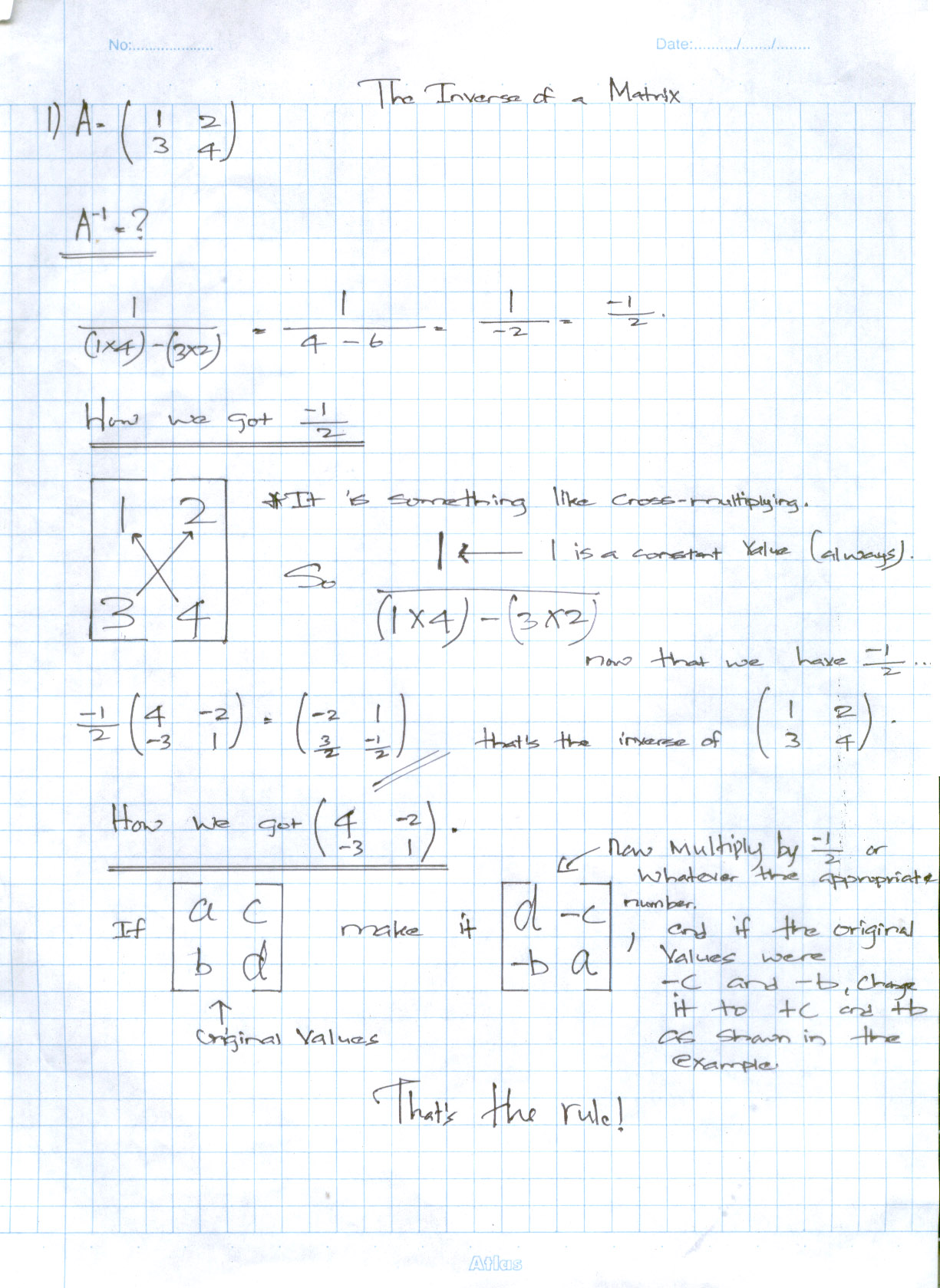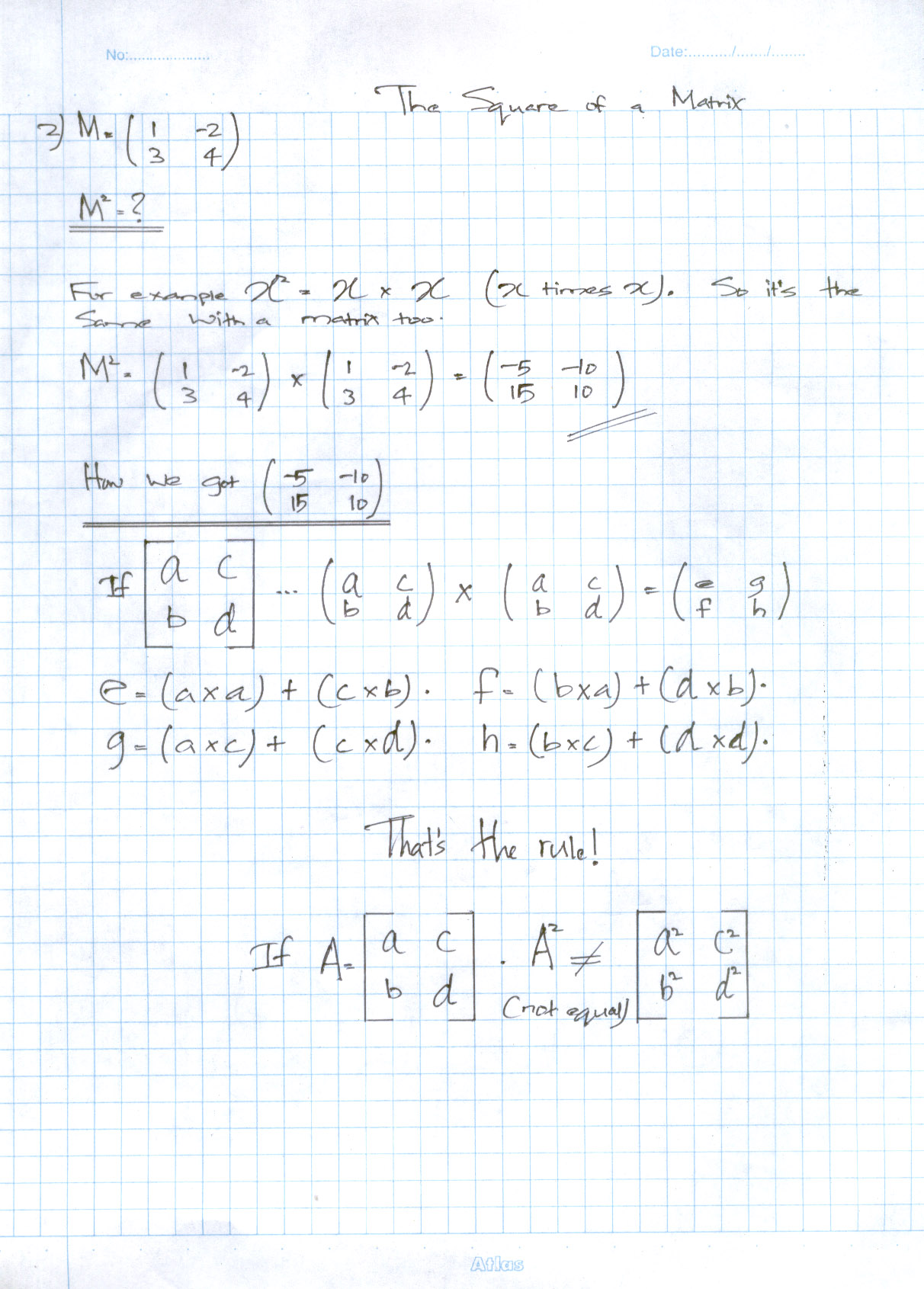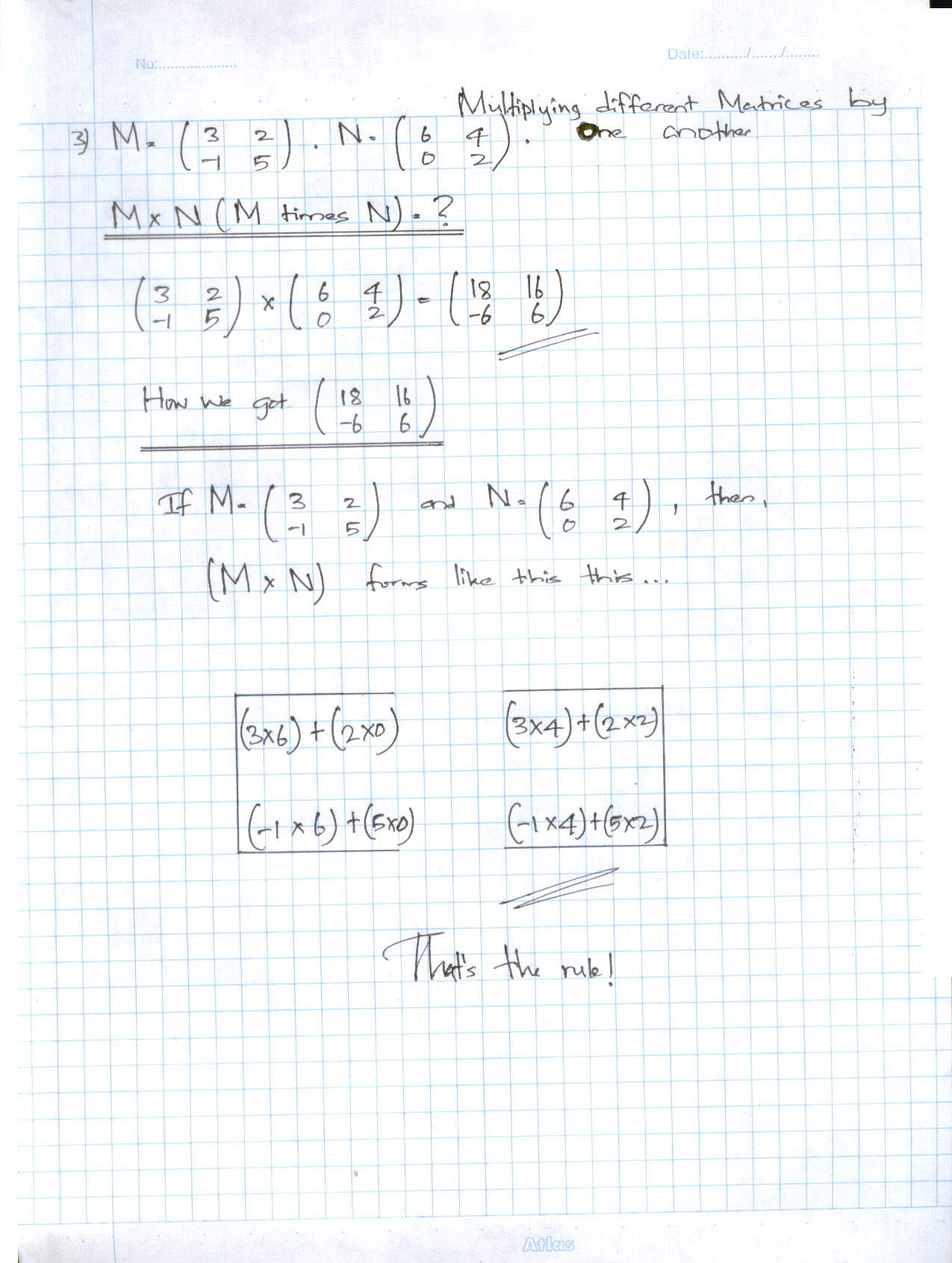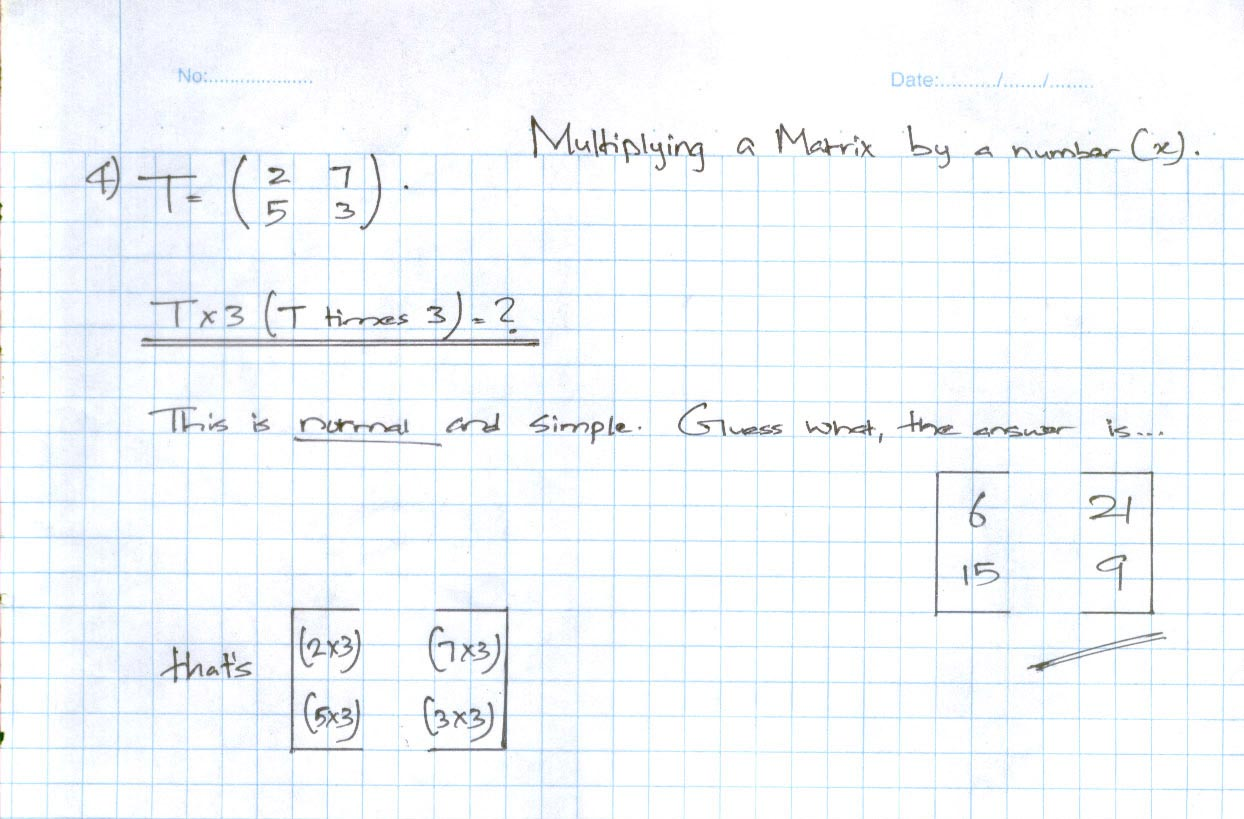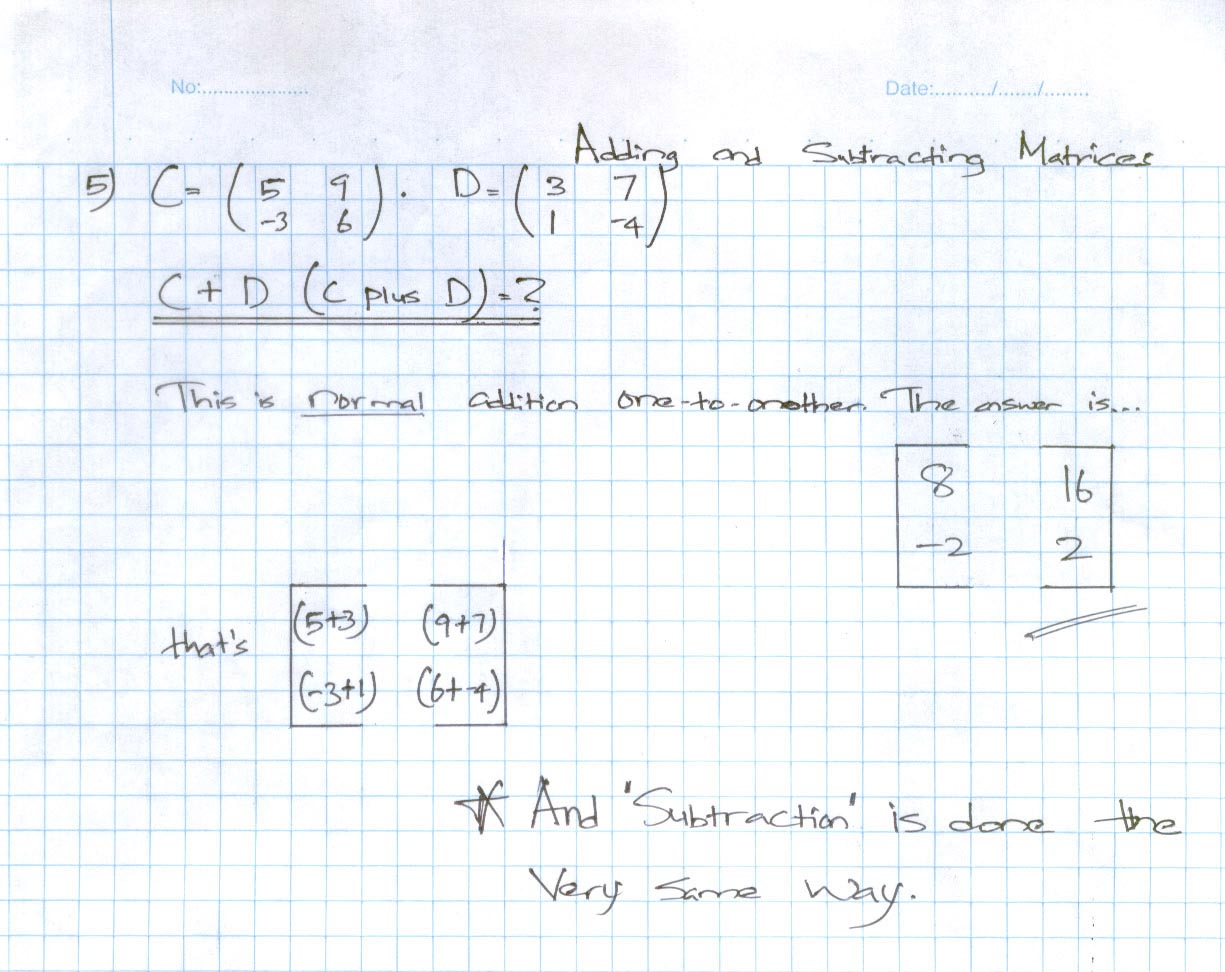1.livelybrowsers says: